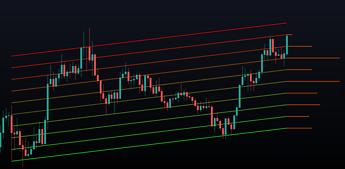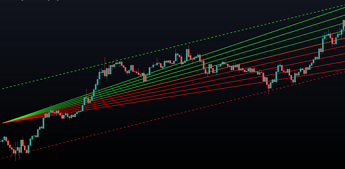# Circular Barplot - Oscillators Sentiment

November 8, 2021

This indicator is an implementation of a circular barplot aiming to return the market sentiment given by multiple normalized oscillators. These include the relative strength index ( RSI ), Stochastic %K (%K), Linear Correlation Oscillator (ROSC), William Percent Range (WPR), Percent Rank (%R), and money flow index ( MFI ).

This indicator is an implementation of a circular barplot aiming to return the market sentiment given by multiple normalized oscillators. These include the relative strength index ( RSI ), Stochastic %K (%K), Linear Correlation Oscillator (ROSC), William Percent Range (WPR), Percent Rank (%R), and money flow index ( MFI ).

The length period of each of these oscillators can be adjusted in the indicator settings.

The label in the center of the circular plot returns the average market sentiment constructed from all the previously mentioned oscillators.

#### Settings

• Width: Circle width.
• Spacing: Determines how close each circle is to the other.
• Thickness: Width of the colored lines.
• Offset: Controls how far the circular barplot left extremity is from the most recent candles.
• Src: Input source of the indicators.

#### Usage

Unlike regular bar charts, circular bar plots display the bars as circle arcs and have the advantage of preserving horizontal and vertical space. A higher arc length would indicate a value closer to the maximal value of the oscillator. Other variations of the circular barplots exist but this variation using the circle arc is particularly appropriate for normalized data.

The indicator can be used as a simple widget giving a quick method to obtain the overall market sentiment of a certain ticker. A dashboard is displayed on the top left of the chart in the event the user wants to see the actual value of the oscillators.

Note that low width or high spacing settings might return unwanted results.

### Other Stories### Linear Regression Histogram

11/23/2021
New Feature
Indicator
Educational

This indicator is inspired by traditional statistical histograms. It will return the number of occurrences of price falling within each interval (bins) of the linear regression channel. This can be useful to highlight zones of interest within a trend.### Linear Regression Fan

11/23/2021
New Feature
Indicator
Educational

This indicator displays a fan using a linear regression fit to the price as a base. All lines are equidistant and are drawn from the first point of the linear regression to the most recent point of the linear regression plus the root-mean-square deviation (RMSD) multiplied by a certain factor.